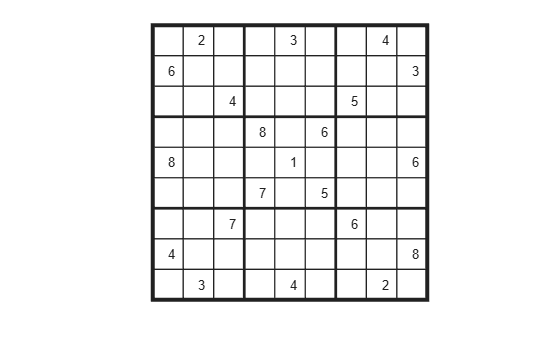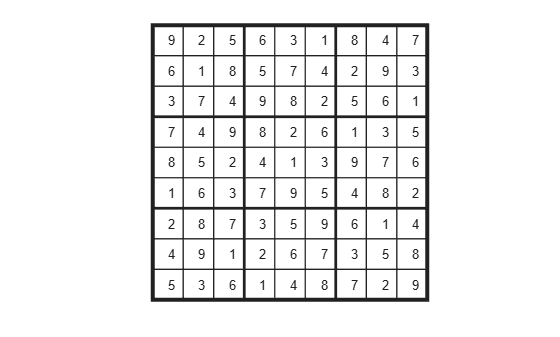# Solve Sudoku Puzzles via Integer Programming: Problem-Based

This example shows how to solve a Sudoku puzzle using binary integer programming. For the solver-based approach, see Solve Sudoku Puzzles via Integer Programming: Solver-Based.

You probably have seen Sudoku puzzles. A puzzle is to fill a 9-by-9 grid with integers from 1 through 9 so that each integer appears only once in each row, column, and major 3-by-3 square. The grid is partially populated with clues, and your task is to fill in the rest of the grid.

### Initial Puzzle

Here is a data matrix `B` of clues. The first row, `B(1,2,2)`, means row 1, column 2 has a clue 2. The second row, `B(1,5,3)`, means row 1, column 5 has a clue 3. Here is the entire matrix `B`.

```B = [1,2,2; 1,5,3; 1,8,4; 2,1,6; 2,9,3; 3,3,4; 3,7,5; 4,4,8; 4,6,6; 5,1,8; 5,5,1; 5,9,6; 6,4,7; 6,6,5; 7,3,7; 7,7,6; 8,1,4; 8,9,8; 9,2,3; 9,5,4; 9,8,2]; drawSudoku(B) % For the listing of this program, see the end of this example.```This puzzle, and an alternative MATLAB® solution technique, was featured in Cleve's Corner in 2009.

There are many approaches to solving Sudoku puzzles manually, as well as many programmatic approaches. This example shows a straightforward approach using binary integer programming.

This approach is particularly simple because you do not give a solution algorithm. Just express the rules of Sudoku, express the clues as constraints on the solution, and then MATLAB produces the solution.

### Binary Integer Programming Approach

The key idea is to transform a puzzle from a square 9-by-9 grid to a cubic 9-by-9-by-9 array of binary values (0 or 1). Think of the cubic array as being 9 square grids stacked on top of each other, where each layer corresponds to an integer from 1 through 9. The top grid, a square layer of the array, has a 1 wherever the solution or clue has a 1. The second layer has a 1 wherever the solution or clue has a 2. The ninth layer has a 1 wherever the solution or clue has a 9.

This formulation is precisely suited for binary integer programming.

The objective function is not needed here, and might as well be a constant term 0. The problem is really just to find a feasible solution, meaning one that satisfies all the constraints. However, for tie breaking in the internals of the integer programming solver, giving increased solution speed, use a nonconstant objective function.

### Express the Rules for Sudoku as Constraints

Suppose a solution $x$ is represented in a 9-by-9-by-9 binary array. What properties does $x$ have? First, each square in the 2-D grid (i,j) has exactly one value, so there is exactly one nonzero element among the 3-D array entries $x\left(i,j,1\right),...,x\left(i,j,9\right)$. In other words, for every $i$ and $j$,

`$\sum _{k=1}^{9}x\left(i,j,k\right)=1.$`

Similarly, in each row $i$ of the 2-D grid, there is exactly one value out of each of the digits from 1 to 9. In other words, for each $i$ and $k$,

`$\sum _{j=1}^{9}x\left(i,j,k\right)=1.$`

And each column $j$ in the 2-D grid has the same property: for each $j$ and $k$,

`$\sum _{i=1}^{9}x\left(i,j,k\right)=1.$`

The major 3-by-3 grids have a similar constraint. For the grid elements $1\le i\le 3$ and $1\le j\le 3$, and for each $1\le k\le 9$,

`$\sum _{i=1}^{3}\sum _{j=1}^{3}x\left(i,j,k\right)=1.$`

To represent all nine major grids, just add 3 or 6 to each $i$ and $j$ index:

$\sum _{i=1}^{3}\sum _{j=1}^{3}x\left(i+U,j+V,k\right)=1,$ where $U,V\phantom{\rule{0.2em}{0ex}}ϵ\phantom{\rule{0.2em}{0ex}}\left\{0,3,6\right\}.$

### Express Clues

Each initial value (clue) can be expressed as a constraint. Suppose that the $\left(i,j\right)$ clue is $m$ for some $1\le m\le 9$. Then $x\left(i,j,m\right)=1$. The constraint $\sum _{k=1}^{9}x\left(i,j,k\right)=1$ ensures that all other $x\left(i,j,k\right)=0$ for $k\ne m$.

### Sudoku in Optimization Problem Form

Create an optimization variable `x` that is binary and of size 9-by-9-by-9.

`x = optimvar('x',9,9,9,'Type','integer','LowerBound',0,'UpperBound',1);`

Create an optimization problem with a rather arbitrary objective function. The objective function can help the solver by destroying the inherent symmetry of the problem.

```sudpuzzle = optimproblem; mul = ones(1,1,9); mul = cumsum(mul,3); sudpuzzle.Objective = sum(sum(sum(x,1),2).*mul);```

Represent the constraints that the sums of `x` in each coordinate direction are one.

```sudpuzzle.Constraints.consx = sum(x,1) == 1; sudpuzzle.Constraints.consy = sum(x,2) == 1; sudpuzzle.Constraints.consz = sum(x,3) == 1;```

Create the constraints that the sums of the major grids sum to one as well.

```majorg = optimconstr(3,3,9); for u = 1:3 for v = 1:3 arr = x(3*(u-1)+1:3*(u-1)+3,3*(v-1)+1:3*(v-1)+3,:); majorg(u,v,:) = sum(sum(arr,1),2) == ones(1,1,9); end end sudpuzzle.Constraints.majorg = majorg;```

Include the initial clues by setting lower bounds of 1 at the clue entries. This setting fixes the value of the corresponding entry to be 1, and so sets the solution at each clued value to be the clue entry.

```for u = 1:size(B,1) x.LowerBound(B(u,1),B(u,2),B(u,3)) = 1; end```

Solve the Sudoku puzzle.

`sudsoln = solve(sudpuzzle)`
```Solving problem using intlinprog. LP: Optimal objective value is 405.000000. Optimal solution found. Intlinprog stopped at the root node because the objective value is within a gap tolerance of the optimal value, options.AbsoluteGapTolerance = 0. The intcon variables are integer within tolerance, options.IntegerTolerance = 1e-05. ```
```sudsoln = struct with fields: x: [9x9x9 double] ```

Round the solution to ensure that all entries are integers, and display the solution.

```sudsoln.x = round(sudsoln.x); y = ones(size(sudsoln.x)); for k = 2:9 y(:,:,k) = k; % multiplier for each depth k end S = sudsoln.x.*y; % multiply each entry by its depth S = sum(S,3); % S is 9-by-9 and holds the solved puzzle drawSudoku(S)```You can easily check that the solution is correct.

### Function to Draw the Sudoku Puzzle

`type drawSudoku`
```function drawSudoku(B) % Function for drawing the Sudoku board % Copyright 2014 The MathWorks, Inc. figure;hold on;axis off;axis equal % prepare to draw rectangle('Position',[0 0 9 9],'LineWidth',3,'Clipping','off') % outside border rectangle('Position',[3,0,3,9],'LineWidth',2) % heavy vertical lines rectangle('Position',[0,3,9,3],'LineWidth',2) % heavy horizontal lines rectangle('Position',[0,1,9,1],'LineWidth',1) % minor horizontal lines rectangle('Position',[0,4,9,1],'LineWidth',1) rectangle('Position',[0,7,9,1],'LineWidth',1) rectangle('Position',[1,0,1,9],'LineWidth',1) % minor vertical lines rectangle('Position',[4,0,1,9],'LineWidth',1) rectangle('Position',[7,0,1,9],'LineWidth',1) % Fill in the clues % % The rows of B are of the form (i,j,k) where i is the row counting from % the top, j is the column, and k is the clue. To place the entries in the % boxes, j is the horizontal distance, 10-i is the vertical distance, and % we subtract 0.5 to center the clue in the box. % % If B is a 9-by-9 matrix, convert it to 3 columns first if size(B,2) == 9 % 9 columns [SM,SN] = meshgrid(1:9); % make i,j entries B = [SN(:),SM(:),B(:)]; % i,j,k rows end for ii = 1:size(B,1) text(B(ii,2)-0.5,9.5-B(ii,1),num2str(B(ii,3))) end hold off end ```